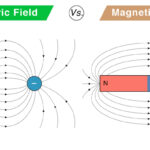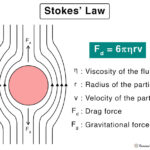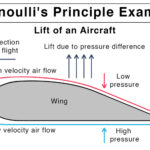Home / Physics / Contact and Non-contact Forces

# Contact and Non-contact Forces

## What are Contact and Non-contact Forces

A contact force is defined as the force between two objects that are in physical contact. For example, kicking a soccer ball is a contact force since physical contact is between the foot and the ball.

A non-contact force is defined as the force between two objects that are not in physical contact. For example, the attraction between the Earth and the Moon is a non-contact force since the two never come in contact. A non-contact force is also called a field force.

## Contact vs. Non-contact Forces

The following table gives the differences between contact and non-contact forces.

## Contact and Non-contact Forces: Types and Examples

### Contact Force

Here is a list of contact forces.

1. Normal Force: Example – The force exerted by the table on a book lying on it.

2. Tension Force: Example – The force experienced by a rope when a person pulls a bucket of water from a well.

3. Friction: Example – The resistance force offered by the floor when a box slides over it.

4. Applied Force: Example – The force with which a person pushes a cart.

5. Air Resistance: Example – The resistance offered by air when a ball falls through it.

6. Spring Force: Example – The force used to measure weight.

### Non-contact Force

There are three types of non-contact force.

1. Gravitational Force: Example – The force of attraction between the Sun and the Earth. Gravity is the force of attraction between the Earth and any object on its surface.

2. Electric Force: ExampleThe force with which electrons are held together by the nucleus.

3. Magnetic Force: Example – The force with which a magnet pulls iron nails.

## How to Find Contact Force

The contact force can be calculated by applying the laws of physics, especially Newton’s Law. For example, the applied force be found by applying Newton’s Second Law.

Suppose an object of mass m is pushed on the floor such that it is moving with an acceleration a. Then, the applied force is given by,

Formula: FA = ma

Article was last reviewed on Wednesday, February 17, 2021

### Related articlesElectric Field vs. Magnetic FieldHooke’s LawStokes’ LawBernoulli’s Principle and Equation